# IBPS Clerk Mains Reasoning Questions 2019 (Day-18) High Level-New Pattern

Dear Aspirants, Reasoning Ability plays a crucial role in Banking and all other competitive exams. To enrich your preparation, here we have provided New Pattern Reasoning Questions for IBPS Clerk Mains. Candidates those who are going to appear in IBPS Clerk Mains can practice these questions daily and make your preparation effective.

[WpProQuiz 4938]

Directions (1 – 3): Study the following information carefully and answer the given questions.

The following is an illustration of Input and rearrangement. Using the illustration answer the question given below.

Step-I: Interchange the Alphabets/Numbers (follow the same pattern as shown in Figure.)
Step-II:
(a) If both letters are Vowel and number is less than 6, then vowels change to next letter in English alphabetical series and add 2 to the number
(b) If both letters are consonant and number is greater than 6 or equal to, then consonants change to the previous letter in English alphabetical series and subtract 3 from the number
(c) If both letters are Vowel and number is greater than 6 or equal to, then vowels change to the previous letter in English alphabetical series and subtract 3 from the number
(d) If both letters are consonant and number is less than 6, then consonants change to next letter in English alphabetical series and add 3 to the number
(e) If there are one vowel and one consonant, then vowel change to next letter and consonant change to the previous letter and add 2 to the number.
(f) If there is single consonant, then consonant change to the previous letter and Subtract 3 from the number.
(g) If there is a single vowel, then vowel change to next letter and add 3 to the number.
Step-III: Follow Both Steps I and II

INPUT: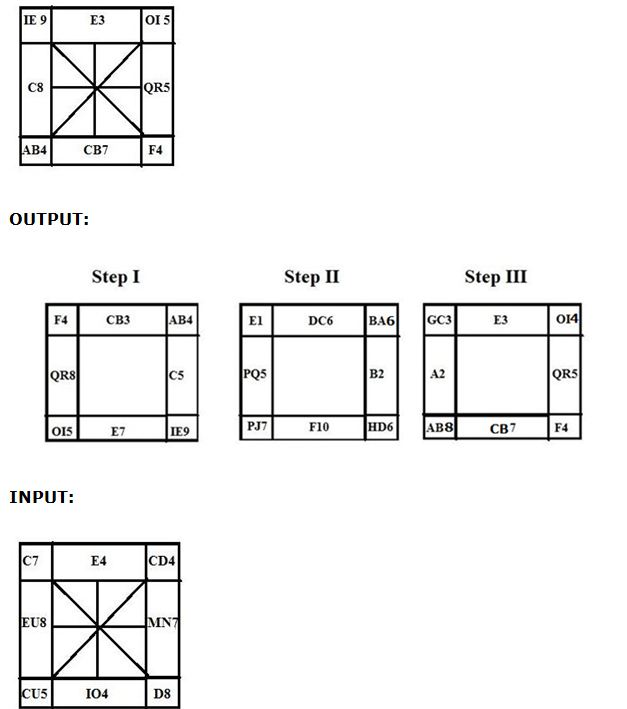1) In Step III, what is the sum of numbers in the first row?

a) 15

b) 11

c) 12

d) 13

e) None of these

2) In Step III, what is the difference between the sum of numbers in the first row and the sum of numbers in the third row?

a) 5

b) 6

c) 3

d) 4

e) None of these

3) In Step II, what is the product of the sum of numbers in the first column and the sum of numbers in the third column?

a) 245

b) 285

c) 275

d) 255

e) 235

Directions (4-7): Study the given information carefully and answer the following questions.

Eight persons A, B, C, D, E, F, G and H are born in a month of July in different years. Their ages are calculated on the same month with respect to 2018.

If it mentioned the person age is considered as last two digits of the person Birth year, then it will be at any sequence. For example, X age is considered as last two digit of Y birth year-1936, and then X age is either 36 or 63.

Note: None of the person born before 1962.

F was born on 1968. The difference between the age of F and B was Five years. C’s age is two less than last two digit of Birth year of B. B’s age is one less than the sum of E’s and C’s age. A is three year elder than C. H’s age is last two digit of A’s birth year. G is four year younger than H. D is born Four year after G.

4) What is the age of D?

a) None of those given as options

b) 14

c) 10

d) 22

e) 38

5) Which of the following is the birth year of G?

a) 1994

b) 2014

c) 2000

d) None of those given as options

e) 2004

6) If I was born between A and C then which of the following can be the birth year of I?

a) 1980

b) None of those given as options

c) 1990

d) 1982

e) 1985

7) What will be the sum of ages of youngest and eldest person in the group?

a) 75 years

b) 65 years

c) 60 years

d) 49 years

e) None of those given as options

Directions (8-10): Study the given information carefully and answer the following questions.

# Means either the hour hand or the minute hand of the clock is at 9

@ Means either the hour hand or the minute hand of the clock is at 8

& Means either the hour hand or the minute hand of the clock is at 4

\$ Means either the hour hand or the minute hand of the clock is at 5

% Means either the hour hand or the minute hand of the clock is at 2

* Means either the hour hand or the minute hand of the clock is at 7

Note: The first symbol represents the hour hand and the second symbol represents the Minute hand. All the given time is considered only in PM.

For example @# means 8.45PM.

8) If the person has an appointment to meet HR at \$#. It takes 45 minutes travel to reach HR office from central bus stand and the person take 25 minutes to reach bus stand from his House. Then when will the person depart from his house to reach on time?

a) None of those give as options

b) &*

c) *&

d) @*

e) \$*

9) Suji walks at 10km speed per hour. She starts walking from point A at %@ and covers a distance of 45km to reach point B. then when will Suji reach at point B?

a) *%

b) %*

c) **

d) None of those given as options

e) &%

10) Shankar is a slow learner, so he takes 25 minutes more than others to complete a task. If Saranya start a task at %\$ and complete the task at &#. Then the same task will complete by Shankar at what time if both the person start a task at a same time?

a) *%

b) *%

c) \$%

d) @%

e) None of those given as options

Directions (1 – 3):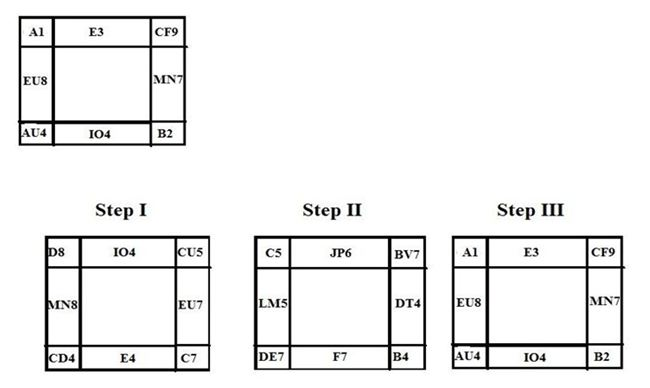Directions (4-7):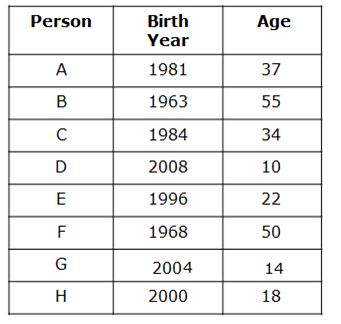Note: None of the person born before 1962.

• If it mentions the person age is considered as last two digits of the person Birth year, then it will be at any sequence. For example, X age is considered as last two digit of Y birth year-1936, and then X age is either 36 or 63.
• F was born on 1968.
• The difference between the age of F and B was Five years. So B can be born in either 1973 or 1963. So we have two possible cases.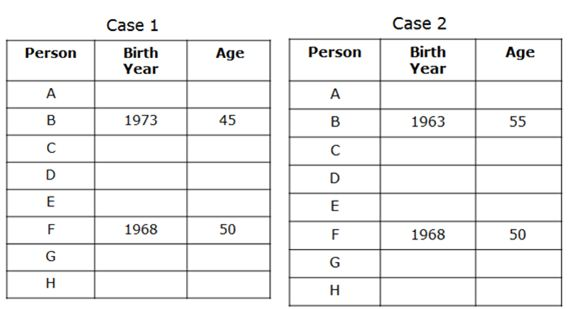• C’s age is two less than last two digit of Birth year of B. Here mention that C’s age is equal to the last two digits of B’s birth year. In both the cases if we take last two digit of B’s birth year in given sequence,then we get C born before 1962. So if we take reverse of last two digits of B’s birth year then it satisfies the given conditions. So C was born on 1983 in Case1 and 1984 in case 2.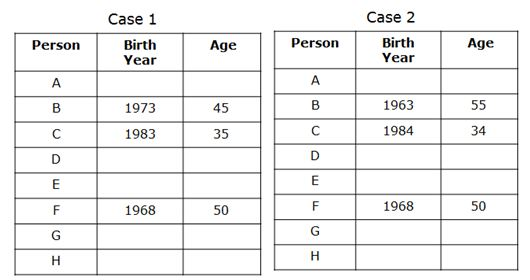• B’s age is one less than the sum of E’s and C’s age. We know that ages of B and C from that we can conclude the age of E as 11 and 22 in case 1 and case 2 respectively.
• A is three year elder than C. Hence the age of A is 38 and 37 in case 1 and case 2 respectively.
• H’s age is last two digit of A’s birth year. It may be 80 or 8 years in case 1 and 18 or 81 in Case 2. But from the note given above H’s age is fixing as 8 and 18 for case 1 and case 2.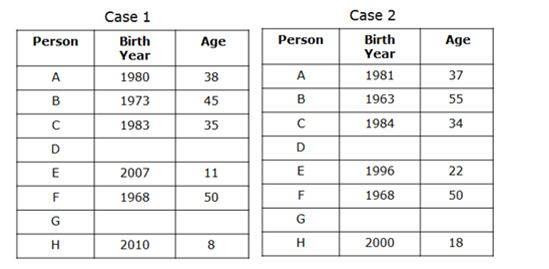• G is four year younger than H. From this G is 4 year old in case 1 and 14 years old Case 2.
• D is born Four year after GHence D is born on 2008 in case 2. But in Case 1 we cannot conclude the birth year of D. Hence Case 1 is eliminated.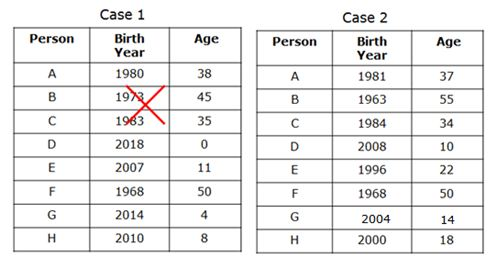Directions (8-10):

\$# – 5.45pm

• It takes 45 minutes travel to reach HR office from central bus stand. So, 5.45- 45 minutes = 5.00pm
• The person takes 25 minutes to reach bus stand from his House.
• So, 5pm -25minutes = 4.35miutes = &*

• She starts walking from point A at %@ = 2.40 minutes
• Suji walk at 10km speed per hour and covers a distance of 45km to reach point B. It means she takes 4hours 30 minutes to reach the point B.
• So, 2.40pm + 4hrs,30minutes = 7.10pm which is *%